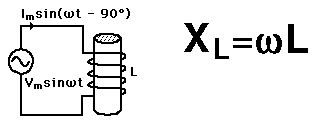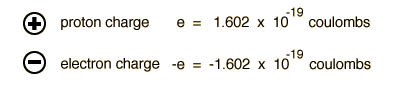# Inductive Reactance

The AC impedance of an inductor is called inductive reactance. It increases with increasing frequency.Index

Capacitance concepts

Inductance concepts
HyperPhysics***** Electricity and Magnetism Go Back

# Electric Charge

The unit of electric charge is the coulomb. Ordinary matter is made up of atoms which have positively charged nuclei and negatively charged electrons surrounding them. Charge is quantized as a multiple of the electron or proton charge:The influence of charges is characterized in terms of the forces between them (Coulomb's law) and the electric field and voltage produced by them. One coulomb of charge is the charge which would flow through a 120 watt lightbulb (120 volts AC) in one second. Two charges of one coulomb each separated by a meter would repel each other with a force of about a million tons!

The rate of flow of electric charge is called electric current and is measured in amperes.

Index

Electromagnetic force
HyperPhysics***** Electricity and Magnetism Go Back